Numismatic Catalog of Venezuela
Cheng's Numismatic
 Jump to code Advanced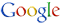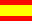Español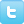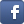## Page Reference

http://www.numismatica.info.ve/en/references/glossary/r.htm

## Glossary beginning by the letter R

A B C D E F G H I J K L M N O P Q R S T U V W X Y Z

Radar serial numberIt's a banknotes which serial number can be read the same forward or backward. This is also known as palindromic number. There are 1 radar banknotes for each 10,000 pieces (based on a 8-digits serie and 90,000,000 pieces), and varies depending the serial interval and amount of digits.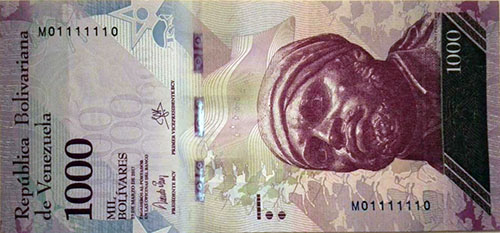(Photograph credits: Victor Ríos. This piece also is 7 digits in-a-row serial number and Binary serie)

Relative amount of low and high serial numberA proposal from this website to calculate the amount of piece to be considered as High serial number or Low serial number based on the amount of issues (oficial or estimated) of a serie. There are three amount of pieces based loosely on known criterias, which maybe used as levels.

The amount of each level includes pieces of higher levels, so to know the amount of each level, it's necessary to substract the amount of pieces from higher levels.

The amount of calculated pieces is not directly proportional to the amount of issues, instead, it's relative with a behavious which is less issues-more pieces, and more issues-less pieces.

The formula is based on a maximum amount of pieces based on 90.000.000 issues, which is:

amount_pieces = issues_amount ( LOG(maximum) / LOG(90000000) ), which is equal to:
amount_pieces = issues_amount ( LOG(maximum) / 7,95424 )
A.- First Level
Based on a known criteria of 10.000 pieces taken from the maximum number of piece using the half amount of 8 digits serie (4 digits). This level without substracting the higher levels amount of pieces can be used to know the general amount of low or high serial number. The relative amount of piece can be calculated using the following formula:

amount_pieces = issues_amount ( LOG(10000) / 7,95424 ), which is also the same to:
amount_pieces = issues_amount ( 4 / 7,95424 ) = issues_amount ( 0,50288 )
B.- Second Level
Based on the criteria of the first 4.000 pieces (1 brick, 4 bundles, 400 straps) and can be calculated using the folowwing formula:

amount_pieces = issues_amount ( LOG(4000) / 7,95424 ), which is equal to:
amount_pieces = issues_amount ( 3,60206 / 7,95424 ) = issues_amount ( 0,45284 )
C.- Third Level
Based on the criteria of the first 1.000 pieces (1 bundle, 10 straps) and can be calculated using the following formula:

amount_pieces = issues_amount ( LOG(1000) / 7,95424 ), which is also the same to:
amount_pieces = issues_amount ( 3 / 7,95424 ) = issues_amount ( 0,37716 )

D.- Fourth Level
Based on the criteria of the first 100 pieces (1 strap) and can be calculated using the following formula:

amount_pieces = issues_amount ( LOG(100) / 7,95424 ), which is also the same to:
amount_pieces = issues_amount ( 3 / 7,95424 ) = issues_amount ( 0,37716 )

Repeater serial numberA banknote with a sequence of digits within the serial number repeats itself, for example "423423", "25252525" and "758758758". The amount of repeater digits depends the length of the serial number, for example:
• Serie of 6 digits: 2 repeat digits and 3 repeat digits
• Serie of 7 digits: None
• Serie of 8 digits: 2 repeat digits and 4 repeat digits (this one the same as Bookend serial number of 4 digits)
• Serie of 9 digits: 3 repeat digits
Example of Repeater serial number (2 digits):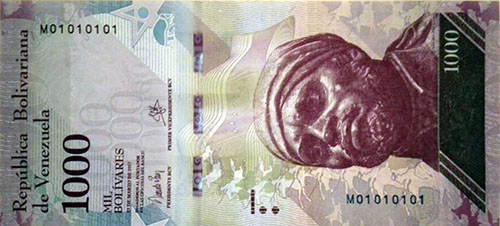(Photograph credits: Victor Ríos. This piece also is Binary serie, Bookend serial number (2 digits), Bookend serial number (4 digits) and Ladder serial number (circular)) Example of Bookend serial number (4 digits):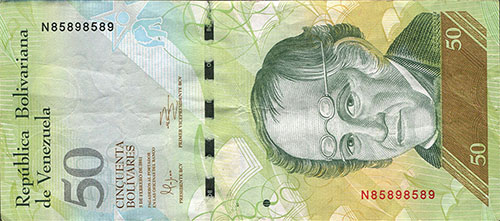(This piece also is Ternary serial number)

Replacement banknoteBanknote that is used to replace defective or low quality printed banknotes. It usually has an special serial number to distinguish among others. Generally the quantity is low, so it is very scarce and the numismatic value is higher than normal banknotes.

In the case of Venezuela, the replacement banknotes started in 1989 with the serie X8 of 1 Bolívar, XX7 of 2 Bolívares and W8 of 5 Bolívares. Generally the series Z8 since 1998 are replacement banknotes. You can see a list of issued replacement banknotes by clicking here.

In the United States, the replacement banknote have a star prefix or suffix of the serial number.

Following is an example of replacement banknote identified as bbcv2bsf-aa01r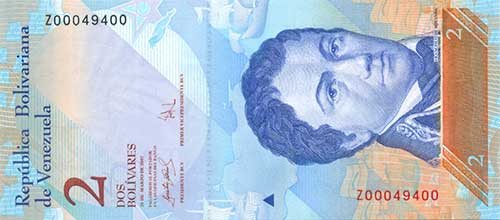(Photograph credits: Numismatic Catalog of Venezuela)

Rotator serial numberA banknote with serial number that can be read the same rotating upside down. The digits of the serial number that allow this are "0", "6", "8" and "9".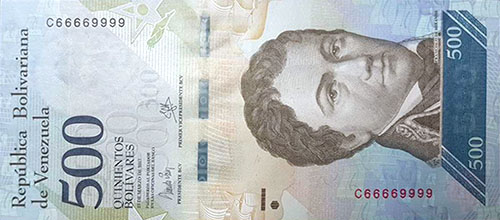(Photograph credits: Maiker Carmona. This piece also is Binary serie and Doubles serial number)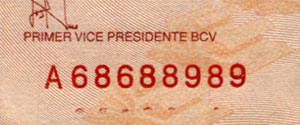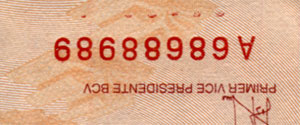(Photograph credits: Ronnie Hobson)